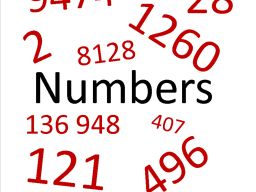# Hard equations

If a<b and c<d and a+b all/2 = c and c+d all/2 = b and if d-a = 60, what is b-c?

s =  20

### Step-by-step explanation:Did you find an error or inaccuracy? Feel free to write us. Thank you!

Tips for related online calculators
Do you have a linear equation or system of equations and looking for its solution? Or do you have a quadratic equation?